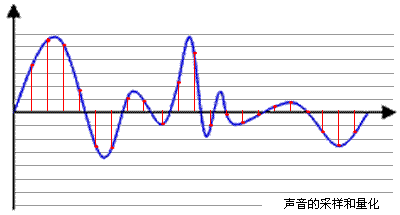#### What are the Basic Concepts of Audio Development?

Publisher: Supplier of LED Display Time: 2022-07-15 17:02 Views: 259

In the audio and video, you need to know such nouns:

(1) Sampling rate (samplerate)

Sampling is to take a value for an analog signal after a period of time. The basis of sampling is determined according to the sampling theorem, that is, the minimum frequency of the sampled signal is not less than twice the bandwidth of the sampled signal, otherwise the original signal will be lost. It can no longer be restored to the original number. Generally, the sampling signal has a larger bandwidth than the sampled signal. For example, the bandwidth of the inter-frequency signal is 20KHZ, and the actual sampling frequency is 44.1KHZ. Sampling is the process of digitizing analog signals, not only Audio needs to be sampled, and all analog signals need to be converted into digital signals that can be represented by 0101 through sampling. The schematic diagram is as follows:Blue represents the analog audio signal, and the red dots represent the sampled quantized values.

The higher the sampling frequency, the denser the red interval, the larger the amount of data used to record this audio signal, and the higher the audio quality.

According to Nyquist theory, as long as the sampling frequency is not lower than twice the highest frequency of the audio signal, the original sound can be restored without loss.

Usually the human ear can hear the sound in the frequency range of about 20Hz to 20kHz. In order to ensure that the sound is not distorted, the sampling frequency should be above 40kHz. Commonly used audio sampling frequencies are: 8kHz, 11.025kHz, 22.05kHz, 16kHz, 37.8kHz, 44.1kHz, 48kHz, 96kHz, 192kHz, etc.

(2) Quantization accuracy (bit width)

In the above picture, each red sampling point needs to be represented by a numerical value. The data type size of this value can be: 4bit, 8bit, 16bit, 32bit, etc. The more the number of bits, the finer the representation. The sound quality is naturally better, and of course, the amount of data will increase exponentially.

Common bit widths are: 8bit or 16bit

(3) Number of channels (channels)

Since the collection and playback of audio can be superimposed, sound can be collected from multiple audio sources at the same time and output to different speakers respectively. Therefore, the number of channels generally indicates the number of sound sources during sound recording or the corresponding number of speakers during playback. .

Mono and Stereo are more common. As the name suggests, the number of channels in the former is 1, and the number of channels in the latter is 2.

(4) Audio frame (frame)

This concept is very important in application development, and many articles on the Internet do not specifically introduce this concept.

Audio is very different from video. Each frame of video is an image. As can be seen from the above Zheng Xuanbo, audio data is streaming, and there is no clear concept of one frame. In practical applications, For the convenience of audio algorithm processing/transmission, the general convention is to take the data volume in units of 2.5ms~60ms as one frame of audio.

This time is called "sampling time". There is no special standard for its length. It is determined according to the needs of the codec and specific applications. We can calculate the size of one audio frame:

Assuming that the audio signal of a channel has a sampling rate of 8kHz, a bit width of 16bit, a frame of 20ms, and two channels, the size of one frame of audio data is:

int size = 8000 x 16bit x 0.02s x 2 = 5120 bit = 640 bytes